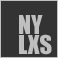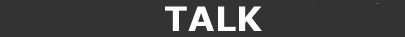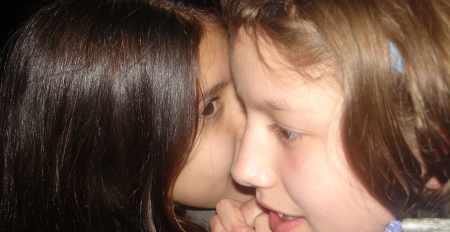Mon May 23 16:14:06 2022EVENTSFREESOFTWAREINSTITUTEPOLITICSJOBSMEMBERS' CORNERMAILING LISTNYLXS Members have a lot to say and share but we don't keep many secrets. Join the Hangout Mailing List and say your peice.## LEARN

2022-05-23 | 2022-04-23 | 2022-03-23 | 2022-02-23 | 2022-01-23 | 2021-12-23 | 2021-11-23 | 2021-10-23 | 2021-09-23 | 2021-08-23 | 2021-07-23 | 2021-06-23 | 2021-05-23 | 2021-04-23 | 2021-03-23 | 2021-02-23 | 2021-01-23 | 2020-12-23 | 2020-11-23 | 2020-10-23 | 2020-09-23 | 2020-08-23 | 2020-07-23 | 2020-06-23 | 2020-05-23 | 2020-04-23 | 2020-03-23 | 2020-02-23 | 2020-01-23 | 2019-12-23 | 2019-11-23 | 2019-10-23 | 2019-09-23 | 2019-08-23 | 2019-07-23 | 2019-06-23 | 2019-05-23 | 2019-04-23 | 2019-03-23 | 2019-02-23 | 2019-01-23 | 2018-12-23 | 2018-11-23 | 2018-10-23 | 2018-09-23 | 2018-08-23 | 2018-07-23 | 2018-06-23 | 2018-05-23 | 2018-04-23 | 2018-03-23 | 2018-02-23 | 2018-01-23 | 2017-12-23 | 2017-11-23 | 2017-10-23 | 2017-09-23 | 2017-08-23 | 2017-07-23 | 2017-06-23 | 2017-05-23 | 2017-04-23 | 2017-03-23 | 2017-02-23 | 2017-01-23 | 2016-12-23 | 2016-11-23 | 2016-10-23 | 2016-09-23 | 2016-08-23 | 2016-07-23 | 2016-06-23 | 2016-05-23 | 2016-04-23 | 2016-03-23 | 2016-02-23 | 2016-01-23 | 2015-12-23 | 2015-11-23 | 2015-10-23 | 2015-09-23 | 2015-08-23 | 2015-07-23 | 2015-06-23 | 2015-05-23 | 2015-04-23 | 2015-03-23 | 2015-02-23 | 2015-01-23 | 2014-12-23 | 2014-11-23 | 2014-10-23

Key: Value:

Key: Value:MESSAGE

color=3D"#ffffff">

background-color: #004065;
color: #FFF;
text-decoration: none;
font-size: 40px;
font-weight: bold;
=20=20=20=20
border: 1px solid #000;
">Perl Weekly

=20=20=20=20

style=3D"border-bottom: 1px solid #ccc;
font-size: 18px;">
Issue #516 - 2021-06-14 - Conference in the Cloud

latest | https://perlweekly.com/archive">archive | edited by anwar.org/">Mohammad S. Anwar

wsletter on the web
, if you prefer.

=20

=20=20=20=20=20=20=20=20

Hi there

=20=20=20=20=20=20=20=20

The highlight of last week was the Conference in the Cloud. It gave=
us the opportunity to meet and greet Perl and Raku fans from across the gl=
obe. On top of that, we got loads of quality talks by experts. Before I tal=
k about anything else, let me thank and congratulate all the organisers and=
volunteers for such a successful event. I am personally impressed with the=
video quality of the live and recorded talks.

=20=20=20=20=20=20=20=20

For me, I find the timing little bit odd as I am in the UK. The day=
started at 6:30pm British Summer Time, which is not ideal. At the end of a=
full day at \$work, there is hardly any energy left to attend the talks liv=
e. But the best part wasthat the recorded talks were available on YouTube i=
mmediately. I find it convenient, as I can watch at my own time. Also I can=
fast-forward if I want to.

=20=20=20=20=20=20=20=20

Please find below my personal favourites in no particular order.

=20=20=20=20=20=20=20=20

1. perlim=
ports or "Where did that symbol come from?" by Olaf Alders

=20=20=20=20=20=20=20=20

2. Local =
dev setup for a complex app using docker-compose by Thomas Klausner

=20=20=20=20=20=20=20=20

3. Introd=
uction to Perl Data Types by William N. Braswell, Jr.

=20=20=20=20=20=20=20=20

4. Rummag=
ing in the clOOset by Curtis Poe

=20=20=20=20=20=20=20=20

5. Life a=
fter Perl (and Raku) by Peter Sergeant

=20=20=20=20=20=20=20=20

6. What's=
new in Perl? by Ricardo Signes

=20=20=20=20=20=20=20=20

7. Perl's=
Amazing Time Machine by Paul Evans

=20=20=20=20=20=20=20=20

8. Valian=
t - Heroic validations for Moo and DBIC classes by John Napiorkowski

=20=20=20=20=20=20=20=20

9. Our sh=
ared vision of Perl by Andrew Solomon

=20=20=20=20=20=20=20=20

10. Cross=
-platform native GUIs: {trade,pay}offs, {integra,distribu}tion by Zaki Mugh=
al

=20=20=20=20=20=20=20=20

There are plenty more to watch later. You can find the complete lis=
t here.

=20=20=20=20=20=20=20=20

=20=20=20=20=20=20=20=20

Announcementsiv>
=20=20=20=20=20=20=20=20

=20=20=20=20=20=20=20=20=20=20=20=20=20=20=20=20
>

yle=3D"
font-size: 18px;
font-weight: bold;
">Proposed RFC for Corinna

r+Corinna+https%3A%2F%2Fgithub.com%2FOvid%2FCor%2Fwiki%2FProposed-RFC by -at-O=
vidPerl via -at-perlweekly">ng" alt=3D"Tweet">

=20
by l.org/users/ovid/">Curtis 'Ovid' Poe (=3D"https://metacpan.org/author/OVID">OVID)
=

Curtis' proposal for creating an RFC for Corinna. What do =

=20=20=20=20=20=20=20=20=20=20=20=20=20=20=20

m/img/curtis_ovid_poe.jpg" title=3D"Curtis 'Ovid' Poe" width=3D"80" />
=20

=20=20=20=20=20=20=20=20=20=20=20=20=20=20=20=20
>

earning-perl-8th-edition/" style=3D"
font-size: 18px;
font-weight: bold;
">Pre-order Learning Perl 8th Edition

ing+Perl+8th+Edition+https%3A%2F%2Fwww.learning-perl.com%2F2021%2F06%2Fpre-=
order-learning-perl-8th-edition%2F by -at-briandfoy_perl via -at-perlweekly"> src=3D"https://perlweekly.com/img/twitter16.png" alt=3D"Tweet">

=20
by l.org/users/brian_d_foy/">brian d foy (=3D"https://metacpan.org/author/BDFOY">BDFOY)
=

=20=20=20=20=20=20=20=20=20=20=20=20=20=20=20

m/img/brian_d_foy.png" title=3D"brian d foy" width=3D"80" />
=20

Articles

=20=20=20=20=20=20=20=20

=20=20=20=20=20=20=20=20=20=20=20=20=20=20=20=20
>

view-56k3" style=3D"
font-size: 18px;
font-weight: bold;
">My TPRCiC 2021 review

review+https%3A%2F%2Fdev.to%2Fthibaultduponchelle%2Ftprcic-2021-review-56k3=
via -at-perlweekly">=3D"Tweet">

=20
by hibaultduponchelle/">Thibault Duponchelle
=

A thorough review of the recently concluded virtual confer=
ence by Thibault.

=20=20=20=20=20=20=20=20=20=20=20=20=20=20=20

m/img/thibault_duponchelle.png" title=3D"Thibault Duponchelle" width=3D"80"=
/>
=20

=20=20=20=20=20=20=20=20=20=20=20=20=20=20=20=20
>

a-example/" style=3D"
font-size: 18px;
font-weight: bold;
">Mojolicious::Plugin::Authentication example

3APlugin%3A%3AAuthentication+example+https%3A%2F%2Fgithub.polettix.it%2FETO=
OBUSY%2F2021%2F06%2F07%2Fmpa-example%2F by -at-polettix via -at-perlweekly">src=3D"https://perlweekly.com/img/twitter16.png" alt=3D"Tweet">

=20
by it">Flavio Poletti (.org/author/POLETTIX">POLETTIX)
=

A practical use case showing the power of Mojolicious::Plu=
gin::Authentication.

=20=20=20=20=20=20=20=20=20=20=20=20=20=20=20

m/img/flavio_poletti.jpg" title=3D"Flavio Poletti" width=3D"80" />
=20

=20=20=20=20=20=20=20=20=20=20=20=20=20=20=20=20
>

e-version/" style=3D"
font-size: 18px;
font-weight: bold;
">use VERSION - but how?

ut+how%3F+https%3A%2F%2Fgithub.polettix.it%2FETOOBUSY%2F2021%2F06%2F12%2Fus=
e-version%2F by -at-polettix via -at-perlweekly">om/img/twitter16.png" alt=3D"Tweet">

=20
by it">Flavio Poletti (.org/author/POLETTIX">POLETTIX)
=

Flavio sharing an interesting use of VERSION.

=20=20=20=20=20=20=20=20=20=20=20=20=20=20=20

m/img/flavio_poletti.jpg" title=3D"Flavio Poletti" width=3D"80" />
=20

=20=20=20=20=20=20=20=20=20=20=20=20=20=20=20=20
>

an/" style=3D"
font-size: 18px;
font-weight: bold;
">EPAN - Exclusive Perl Archive Nook

e+Perl+Archive+Nook+https%3A%2F%2Fgithub.polettix.it%2FETOOBUSY%2F2021%2F06=
%2F13%2Fepan%2F by -at-polettix via -at-perlweekly">y.com/img/twitter16.png" alt=3D"Tweet">

=20
by it">Flavio Poletti (.org/author/POLETTIX">POLETTIX)
=

Flavio's 10-year-old code is finally out in public.

=20=20=20=20=20=20=20=20=20=20=20=20=20=20=20

m/img/flavio_poletti.jpg" title=3D"Flavio Poletti" width=3D"80" />
=20

=20=20=20=20=20=20=20=20=20=20=20=20=20=20=20=20
>

pment-history-4afm" style=3D"
font-size: 18px;
font-weight: bold;
">Visualization of perl development history

f+perl+development+history+https%3A%2F%2Fdev.to%2Fgugod%2Fvisualization-of-=

=20
by Kang-min Liu
<=
p style=3D"font-size: 16px">
Kang-min shared a 10-year review of perl5.git.

=20=20=20=20=20=20=20=20=20=20=20=20=20=20=20

=20=20=20=20=20=20=20=20=20=20=20
=20

=20=20=20=20=20=20=20=20=20=20=20=20=20=20=20=20
>

jolicious-routes-to-their-own-module/" style=3D"
font-size: 18px;
font-weight: bold;
">Moving Perl Mojolicious routes to their own module

olicious+routes+to+their+own+module+https%3A%2F%2Fphoenixtrap.com%2F2021%2F=
06%2F08%2Fmoving-perl-mojolicious-routes-to-their-own-module%2F via -at-perlwe=
ekly"><=
/a>

=20
by
rap.com/">Mark Gardner

=3D"font-size: 16px">
Mark experimenting with Mojolicious routes. Nice work.

=20=20=20=20=20=20=20=20=20=20=20=20=20=20=20

m/img/mark_gardner.jpeg" title=3D"Mark Gardner" width=3D"80" />
=20

Slides

=20=20=20=20=20=20=20=20

=20=20=20=20=20=20=20=20=20=20=20=20=20=20=20=20
>

ogging-outside-the-bubble-perl-talk/" style=3D"
font-size: 18px;
font-weight: bold;
">Slides from "Blogging Outside the Bubble" Per=
l talk

Blogging+Outside+the+Bubble%22+Perl+talk+https%3A%2F%2Fphoenixtrap.com%2F20=
21%2F06%2F09%2Fslides-from-blogging-outside-the-bubble-perl-talk%2F via -at-pe=
rlweekly">t">

=20
by rap.com/">Mark Gardner

=3D"font-size: 16px">
Mark's slides from a recent talk he gave at CiC 2021.

=20=20=20=20=20=20=20=20=20=20=20=20=20=20=20

m/img/mark_gardner.jpeg" title=3D"Mark Gardner" width=3D"80" />
=20

Perl Weekly Chal=
lenge

=20=20=20=20=20=20=20=20

enge.org/">The Weekly Challenge by M=
win prize money of \$50 Amazon vouchers by participating in the weekly chal=
lenge. We pick one winner at the end of the month from all the contributor=
s during that month. The monthly prize is kindly sponsored by Peter Sergean=
t of PerlCareers.

=20=20=20=20=20=20=20=20

=20=20=20=20=20=20=20=20=20=20=20=20=20=20=20=20
>

challenge-117" style=3D"
font-size: 18px;
font-weight: bold;
">The Weekly Challenge - 117

lenge+-+117+https%3A%2F%2Fperlweeklychallenge.org%2Fblog%2Fperl-weekly-chal=
lenge-117 by -at-cpan_author via -at-perlweekly">om/img/twitter16.png" alt=3D"Tweet">

=20
<=
p style=3D"font-size: 16px">
Welcome to a new week with a couple of fun tasks "Missing =
Row" and "Find Possible Paths". If you are new to the weekly challenge then=
FAQ page.

=20=20=20=20=20=20=20=20=20=20=20=20=20=20=20

=20

=20=20=20=20=20=20=20=20=20=20=20=20=20=20=20=20
>

nge-116" style=3D"
font-size: 18px;
font-weight: bold;
">RECAP - The Weekly Challenge - 116

kly+Challenge+-+116+https%3A%2F%2Fperlweeklychallenge.org%2Fblog%2Frecap-ch=
allenge-116 by -at-cpan_author via -at-perlweekly">.com/img/twitter16.png" alt=3D"Tweet">

=20
<=
p style=3D"font-size: 16px">
Enjoy a quick recap of last week's contributions by Team P=
WC dealing with the "Number Sequence" and "Sum of Squares" tasks in Perl an=
d Raku. You will find plenty of solutions to keep you busy.

=20=20=20=20=20=20=20=20=20=20=20=20=20=20=20

=20

=20=20=20=20=20=20=20=20=20=20=20=20=20=20=20=20
>

-challenge-116/" style=3D"
font-size: 18px;
font-weight: bold;
">Perl Weekly Challenge 116

llenge+116+https%3A%2F%2Faaronreidsmith.github.io%2Fblog%2Fperl-weekly-chal=
lenge-116%2F via -at-perlweekly">r16.png" alt=3D"Tweet">

=20
by Aaron Smith
style=3D"font-size: 16px">
Aaron's use of Raku's power makes the task look so simple.=
Incredible.

=20=20=20=20=20=20=20=20=20=20=20=20=20=20=20

=20=20=20=20=20=20=20=20=20=20=20
=20

=20=20=20=20=20=20=20=20=20=20=20=20=20=20=20=20
>

nge/week-116-1.html" style=3D"
font-size: 18px;
font-weight: bold;
">Perl Weekly Challenge 116: Number Sequence

llenge+116%3A+Number+Sequence+https%3A%2F%2Fabigail.github.io%2FHTML%2FPerl=

=20
by Abigail

le=3D"font-size: 16px">
Abigail's solution was hard to follow initially, but after=
the second read it become obvious. Thanks for sharing.

=20=20=20=20=20=20=20=20=20=20=20=20=20=20=20

=20=20=20=20=20=20=20=20=20=20=20
=20

=20=20=20=20=20=20=20=20=20=20=20=20=20=20=20=20
>

nge/week-116-2.html" style=3D"
font-size: 18px;
font-weight: bold;
">Perl Weekly Challenge 116: Sum of Squares

llenge+116%3A+Sum+of+Squares+https%3A%2F%2Fabigail.github.io%2FHTML%2FPerl-=

=20
by Abigail

le=3D"font-size: 16px">
For this task, Abigail split the task to make it more easy=
to understand. Cool attempt.

=20=20=20=20=20=20=20=20=20=20=20=20=20=20=20

=20=20=20=20=20=20=20=20=20=20=20
=20

=20=20=20=20=20=20=20=20=20=20=20=20=20=20=20=20
>

21/06/13" style=3D"
font-size: 18px;
font-weight: bold;
">Evolving a Sequence with a Functional Genome: The Weekl=
y Challenge 116

ence+with+a+Functional+Genome%3A+The+Weekly+Challenge+116+http%3A%2F%2Fwww.=
rabbitfarm.com%2Fcgi-bin%2Fblosxom%2Fperl%2F2021%2F06%2F13 via -at-perlweekly"=
>

=20
=

I love the use of genetics to solve the task. My favourite=
this week. I will revisit it later.

=20=20=20=20=20=20=20=20=20=20=20=20=20=20=20

=20

=20=20=20=20=20=20=20=20=20=20=20=20=20=20=20=20
>

style=3D"
font-size: 18px;
font-weight: bold;
">Sequential Squares with Raku and Perl

res+with+Raku+and+Perl+https%3A%2F%2Fraku-musings.com%2Fsequential-squares.=
html via -at-perlweekly"> alt=3D"Tweet">

=20
by Arne Sommer
style=3D"font-size: 16px">
Arne's bonus solution is really nice and his workaround ha=
ck used in Perl solution is cool. Thanks for sharing.

=20=20=20=20=20=20=20=20=20=20=20=20=20=20=20

=20=20=20=20=20=20=20=20=20=20=20
=20

=20=20=20=20=20=20=20=20=20=20=20=20=20=20=20=20
>

ing-sequet-numbers/" style=3D"
font-size: 18px;
font-weight: bold;
">Shhh=E2=80=A6 We=E2=80=99re Squaring Sequet Numbers

e%E2%80%99re+Squaring+Sequet+Numbers+https%3A%2F%2Fcolincrain.com%2F2021%2F=

=20
by Colin Crain
style=3D"font-size: 16px">
Colin 'sblog is always full of interesting ideas. I loved =
his task analysis. Keep it going.

=20=20=20=20=20=20=20=20=20=20=20=20=20=20=20

=20=20=20=20=20=20=20=20=20=20=20
=20

=20=20=20=20=20=20=20=20=20=20=20=20=20=20=20=20
>

are-perl-weekly-challenge-116.html" style=3D"
font-size: 18px;
font-weight: bold;
">Hip To Be Square: Perl Weekly Challenge #116

e%3A+Perl+Weekly+Challenge+%23116+https%3A%2F%2Fjacoby.github.io%2F2021%2F0=
6%2F08%2Fhip-to-be-square-perl-weekly-challenge-116.html by -at-jacobydave via=
-at-perlweekly">Tweet">

=20
by t.blogspot.com/">Dave Jacoby (//metacpan.org/author/JACOBY">JACOBY)
=

Dave's use of a substr hack recursively to solve the task =

=20=20=20=20=20=20=20=20=20=20=20=20=20=20=20

m/img/dave_jacoby.jpg" title=3D"Dave Jacoby" width=3D"80" />
=20

=20=20=20=20=20=20=20=20=20=20=20=20=20=20=20=20
>

c116-number-sequence/" style=3D"
font-size: 18px;
font-weight: bold;
">PWC116 - Number Sequence

+Sequence+https%3A%2F%2Fgithub.polettix.it%2FETOOBUSY%2F2021%2F06%2F09%2Fpw=
c116-number-sequence%2F by -at-polettix via -at-perlweekly">erlweekly.com/img/twitter16.png" alt=3D"Tweet">

=20
by it">Flavio Poletti (.org/author/POLETTIX">POLETTIX)
=

I enjoyed Raku solution more as it reminds me how I starte=
d using Raku. Brilliant job.

=20=20=20=20=20=20=20=20=20=20=20=20=20=20=20

m/img/flavio_poletti.jpg" title=3D"Flavio Poletti" width=3D"80" />
=20

=20=20=20=20=20=20=20=20=20=20=20=20=20=20=20=20
>

c116-sum-of-squares/" style=3D"
font-size: 18px;
font-weight: bold;
">PWC116 - Sum of Squares

+Squares+https%3A%2F%2Fgithub.polettix.it%2FETOOBUSY%2F2021%2F06%2F10%2Fpwc=
116-sum-of-squares%2F by -at-polettix via -at-perlweekly">lweekly.com/img/twitter16.png" alt=3D"Tweet">

=20
by it">Flavio Poletti (.org/author/POLETTIX">POLETTIX)
=

Flavio's commentary on Raku features in interesting. Once =
again, the Raku solution wins the race for me. Thanks for sharing.

=20=20=20=20=20=20=20=20=20=20=20=20=20=20=20

m/img/flavio_poletti.jpg" title=3D"Flavio Poletti" width=3D"80" />
=20

=20=20=20=20=20=20=20=20=20=20=20=20=20=20=20=20
>

ub/tree/master/challenge-116/james-smith" style=3D"
font-size: 18px;
font-weight: bold;
">Perl Weekly Challenge #116

llenge+%23116+https%3A%2F%2Fgithub.com%2Fdrbaggy%2Fperlweeklychallenge-club=

=20
by James Smith
style=3D"font-size: 16px">
James found the second task easier than than the first and=
he proved it with his solutions. Oops.

=20=20=20=20=20=20=20=20=20=20=20=20=20=20=20

=20=20=20=20=20=20=20=20=20=20=20
=20

=20=20=20=20=20=20=20=20=20=20=20=20=20=20=20=20
>

l-weekly-challenge-116-number-sequence-and-sum-of-squares.html" style=3D"
font-size: 18px;
font-weight: bold;
">Perl Weekly Challenge 116: Number Sequence and Sum of S=
quares

llenge+116%3A+Number+Sequence+and+Sum+of+Squares+http%3A%2F%2Fblogs.perl.or=
g%2Fusers%2Flaurent_r%2F2021%2F06%2Fperl-weekly-challenge-116-number-sequen=

=20
by Laurent Rosenfeld
=

Laurent's blog is always very special as there are plenty =
of ideas to explore. Please check out the Raku tricks.

=20=20=20=20=20=20=20=20=20=20=20=20=20=20=20

=20=20=20=20=20=20=20=20=20=20=20
=20

=20=20=20=20=20=20=20=20=20=20=20=20=20=20=20=20
>

Challenge116.html" style=3D"
font-size: 18px;
font-weight: bold;
">Perl Weekly Challenge 116: numbers

llenge+116%3A+numbers+https%3A%2F%2Ffluca1978.github.io%2F2021%2F06%2F08%2F=

=20
by .blogspot.com">Luca Ferrari

tyle=3D"font-size: 16px">
Luca's verbose solution is so simple to follow. Thanks for=
sharing.

=20=20=20=20=20=20=20=20=20=20=20=20=20=20=20

=20=20=20=20=20=20=20=20=20=20=20
=20

=20=20=20=20=20=20=20=20=20=20=20=20=20=20=20=20
>

=3D"
font-size: 18px;
font-weight: bold;
">Perl Weekly Challenge 116

llenge+116+https%3A%2F%2Fwlmb.github.io%2F2021%2F06%2F11%2FPWC116%2F via -at-p=
erlweekly">et">

=20
by W Luis Mochan
=

Luis' one-liner is the winner for me this week. Well done.

=20=20=20=20=20=20=20=20=20=20=20=20=20=20=20

=20=20=20=20=20=20=20=20=20=20=20
=20

=20=20=20=20=20=20=20=20=20=20=20=20=20=20=20=20
>

eekly_Challenge_116__Square_Sequence.html" style=3D"
font-size: 18px;
font-weight: bold;
">Perl Weekly Challenge 116: Square Sequence

llenge+116%3A+Square+Sequence+https%3A%2F%2Fblog.firedrake.org%2Farchive%2F=
2021%2F06%2FPerl_Weekly_Challenge_116__Square_Sequence.html via -at-perlweekly=
">

=20
by edrake.org/">Roger Bell West (//metacpan.org/author/FIREDRAKE">FIREDRAKE)
=

Roger figured out an interesting output from one of the gi=
ven example. Good catch.

=20=20=20=20=20=20=20=20=20=20=20=20=20=20=20

=20=20=20=20=20=20=20=20=20=20=20
=20

=20=20=20=20=20=20=20=20=20=20=20=20=20=20=20=20
>

-1mf7" style=3D"
font-size: 18px;
font-weight: bold;
">Weekly Challenge 116

e+116+https%3A%2F%2Fdev.to%2Fsimongreennet%2Fweekly-challenge-116-1mf7 via =
-at-perlweekly">weet">

=20
by Simon Green
style=3D"font-size: 16px">
Simon shared an interesting story about numbers. Thanks fo=
r sharing the story and solutions.

=20=20=20=20=20=20=20=20=20=20=20=20=20=20=20

=20=20=20=20=20=20=20=20=20=20=20
=20

Perl Tutorialiv>
=20=20=20=20=20=20=20=20

A section for newbies and for peop=
le who need some refreshing of their Perl knowledge. If you have questions =
or suggestions about the articles, let me know and I'll try to make the nec=
essary changes. The included articles are from the aven.com/perl-tutorial">Perl Maven Tutorial and are part of the =3D"https://leanpub.com/perl-maven">Perl Maven eBook.

=20=20=20=20=20=20=20=20

=20=20=20=20=20=20=20=20=20=20=20=20=20=20=20=20
>

font-size: 18px;
font-weight: bold;
">Create a lexical warning that can be turned on and off =
with the "warnings" pragma.

l+warning+that+can+be+turned+on+and+off+with+the+%22warnings%22+pragma.+htt=
ps%3A%2F%2Fperlmaven.com%2Fcreate-warning by -at-szabgab via -at-perlweekly"> src=3D"https://perlweekly.com/img/twitter16.png" alt=3D"Tweet">

=20
by om/">Gabor Szabo (rg/author/SZABGAB">SZABGAB)

tyle=3D"font-size: 16px">
=20=20=20=20=20=20=20=20=20=20=20=20=20=20=20=20=20

=20=20=20=20=20=20=20=20=20=20=20=20=20=20=20

m/img/gabor_szabo.png" title=3D"Gabor Szabo" width=3D"80" />
=20

Videos

=20=20=20=20=20=20=20=20

=20=20=20=20=20=20=20=20=20=20=20=20=20=20=20=20
>

font-size: 18px;
font-weight: bold;
">Conference in the Cloud! A Perl and Raku Conf

%2Fvideos via -at-perlweekly">.png" alt=3D"Tweet">

=20=20=20=20=20=20=20=20=20=20=20=20=20=20=20=20=20

=20=20=20=20=20=20=20=20=20=20=20=20=20=20=20

=20=20=20=20=20=20=20=20=20=20=20
=20

Rakudo

=20=20=20=20=20=20=20=20

=20=20=20=20=20=20=20=20=20=20=20=20=20=20=20=20
>

g-funky/" style=3D"
font-size: 18px;
font-weight: bold;
">2021.23 Going Funky!

unky%21+https%3A%2F%2Frakudoweekly.blog%2F2021%2F06%2F07%2F2021-23-going-fu=
nky%2F via -at-perlweekly">g" alt=3D"Tweet">

=20
by Elizabeth Mattijsen (=3D"https://metacpan.org/author/ELIZABETH">ELIZABETH) =

=20=20=20=20=20=20=20=20=20=20=20=20=20=20=20=20=20

=20=20=20=20=20=20=20=20=20=20=20=20=20=20=20

m/img/elizabeth_mattijsen.png" title=3D"Elizabeth Mattijsen" width=3D"80" />
=20

Weekly collectio=
ns

=20=20=20=20=20=20=20=20

=20=20=20=20=20=20=20=20=20=20=20=20=20=20=20=20
>

font-size: 18px;
font-weight: bold;

1. 2021-06-14 Gabor Szabo Subject: [Learn] [Perlweekly] #516 - Conference in the Cloud
2. 2021-06-21 Gabor Szabo Subject: [Learn] [Perlweekly] #517 - Commitment and habit

NYLXS are Do'ers and the first step of Doing is Joining! Join NYLXS and make a difference in your community today!

=20=20=20=20

=20=20=20=20

=20=20=20=20
=20=20=20=20

=20=20=20=20

=20=20=20=20

=20=20=20=20

=20=20=20=20

=20=20=20=20

=20=20=20=20

=20=20=20=20
=20=20=20=20

=20=20=20=20

=20=20=20=20
=20=20=20=20

=20=20=20=20

=20=20=20=20

=20=20=20=20

=20=20=20=20

=20=20=20=20

=20=20=20=20

=20=20=20=20

=20=20=20=20

=20=20=20=20

=20=20=20=20

=20=20=20=20

=20=20=20=20

=20=20=20=20

=20=20=20=20

=20=20=20=20

=20=20=20=20

=20=20=20=20

=20=20=20=20
=20=20=20=20

=20=20=20=20

=20=20=20=20
=20=20=20=20

=20=20=20=20

=20=20=20=20
=20=20=20=20

=20=20=20=20

=20=20=20=20
=20=20=20=20

=20=20=20=20